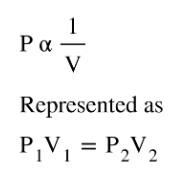# Problem: A large scuba tank (Figure 9.16) with a volume of 18 L is rated for a pressure of 220 bar. The tank is filled at 20 °C and contains enough air to supply 1860 L of air to a diver at a pressure of 2.37 atm (a depth of 45 feet). Was the tank filled to capacity at 20 °C?

###### FREE Expert Solution
• According to Boyle's Law, at constant T, Pressure is inversely proportional to the Volume which means as pressure increases, V decreases:• The tank with 18 L was said to be rated with a pressure of 220 bar, so P2 calculated must not exceed 220 bar
83% (284 ratings)###### Problem Details

A large scuba tank (Figure 9.16) with a volume of 18 L is rated for a pressure of 220 bar. The tank is filled at 20 °C and contains enough air to supply 1860 L of air to a diver at a pressure of 2.37 atm (a depth of 45 feet). Was the tank filled to capacity at 20 °C?